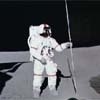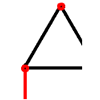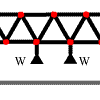#### You may also like### Lunar Leaper

Gravity on the Moon is about 1/6th that on the Earth. A pole-vaulter 2 metres tall can clear a 5 metres pole on the Earth. How high a pole could he clear on the Moon?### Bridge Builder

In this short problem we investigate the tensions and compressions in a framework made from springs and ropes.### More Bridge Building

Which parts of these framework bridges are in tension and which parts are in compression?

# Wobbler

##### Age 16 to 18Challenge Level

For this question you need to know the centres of mass of the hemisphere and the cone and they are calculated below (you will need to fill in some of the intermediate steps for yourself). You can use the positions of the centres of mass given here.

In order to find the centre of mass of the hemisphere consider a cylindrical element of thickness $\delta x$ at distance $x$ from the centre of the base $O$. Then the radius of this slice is $\sqrt{a^2 - x^2}$. The position of the centre of mass is found by taking moments about $O$ and summing these moments for all the 'slices' by integrating: $${2\over 3}w\pi a^3\bar{x}= \int_0^a x \times w\pi (a^2-x^2)dx$$ which gives $$\bar{x}={3a\over 8}.$$ Similarly to find the centre of mass of the uniform cone, a distance $\bar{x}$ along the axis of symmetry from the vertex $V$, consider cylindrical elements of thickness $\delta x$ and radius ${ax\over h}$ (from similar triangles). The position of the centre of mass is again found by taking moments, in this case about $V$: $${1\over 3}w\pi a^2h\bar{x} = \int_0^h x\times w\pi {a^2x^2\over h^2}dx$$ which gives $$\bar{x}= {3h\over 4}.$$